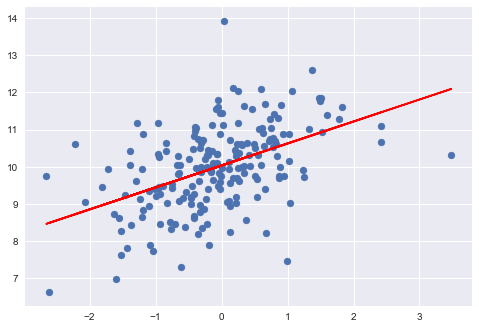# Basic Principles¶

Show different ways to present statistical data.

This script is written in MATLAB or IPpython style, to show how best to use Python interactively. Note than in IPython, the show() commands are automatically generated. The examples contain:

• scatter plots
• histograms
• KDE
• errorbars
• boxplots
• probplots
• cumulative density functions
• regression fits

Author: thomas haslwanter, March-2015

First, import the libraries that you are going to need. You could also do that later, but it is better style to do that at the beginning. pylab imports the numpy, scipy, and matplotlib.pyplot libraries into the current environment

In :
%pylab inline

import scipy.stats as stats
import seaborn as sns
sns.set_context('notebook')
sns.set_style('darkgrid')

Populating the interactive namespace from numpy and matplotlib

In :
# Generate data that are normally distributed
x = randn(50)


## Scatter plot¶

In :
plot(x,'.')
title('Scatter Plot')
xlabel('X')
ylabel('Y')
draw()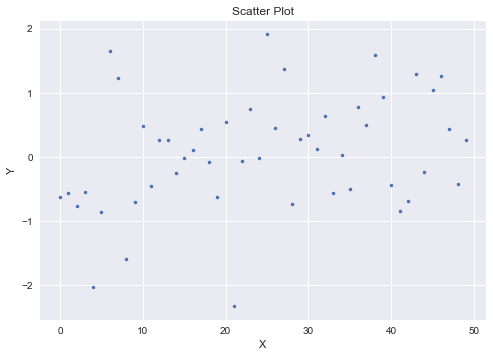## Histogram¶

In :
hist(x)
xlabel('Data Values')
ylabel('Frequency')
title('Histogram, default settings')

Out:
<matplotlib.text.Text at 0x24320fd3550>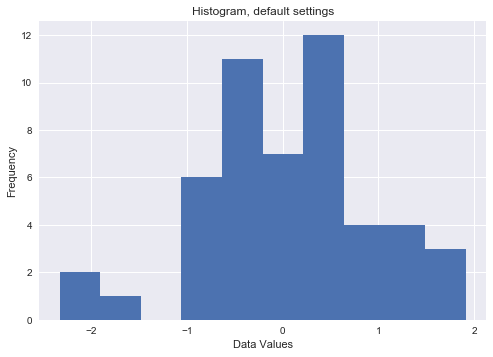In :
x = randn(1000)

In :
hist(x,25)
xlabel('Data Values')
ylabel('Frequency')
title('Histogram, 25 bins')

Out:
<matplotlib.text.Text at 0x2432172c978>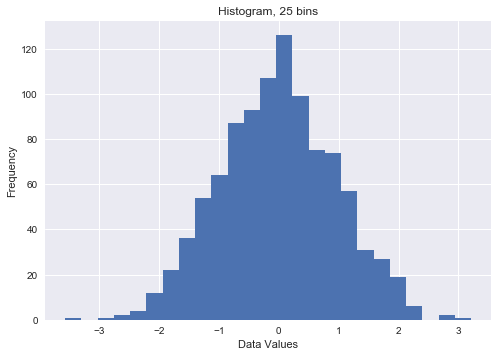### KDE¶

Kernel Density Estimation (KDE)

In :
import seaborn as sns
sns.kdeplot(x)
xlabel('Data Values')
ylabel('Density')

Out:
<matplotlib.text.Text at 0x243215c6e10>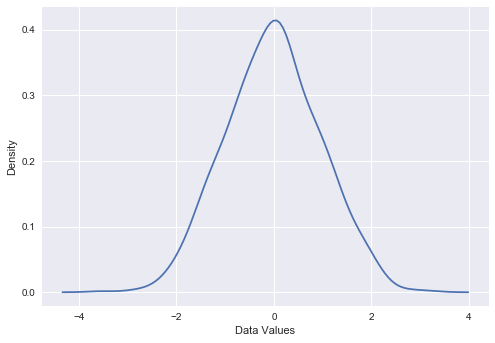## Cumulative probability density¶

In :
numbins = 20
cdf = stats.cumfreq(x,numbins)
plot(cdf)
xlabel('Data Values')
ylabel('Cumulative Frequency')
title('Cumulative probablity density function')

Out:
<matplotlib.text.Text at 0x24320f4f9b0>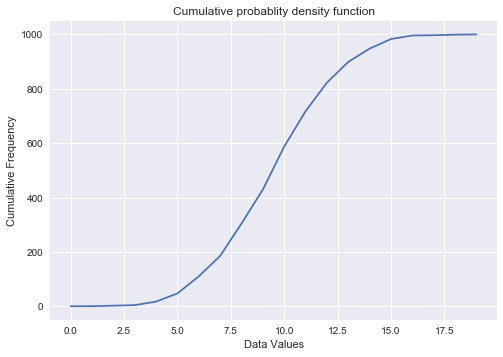## Boxplot¶

In :
# The error bars indicate 1.5* the inter-quartile-range (IQR), and the box consists of the
# first, second (middle) and third quartile
boxplot(x, sym='o')
title('Boxplot')
ylabel('Values')

Out:
<matplotlib.text.Text at 0x24320fe1550>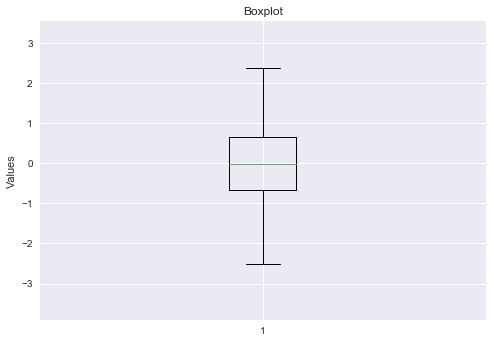In :
boxplot(x, vert=False, sym='*')
title('Boxplot, horizontal')
xlabel('Values')

Out:
<matplotlib.text.Text at 0x24321737748>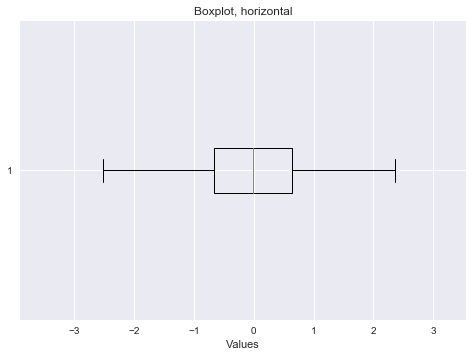## Errorbars¶

In :
x = arange(5)
y = x**2
errorBar = x/2
errorbar(x,y, yerr=errorBar, fmt='o', capsize=5, capthick=3)

plt.xlabel('Data Values')
plt.ylabel('Measurements')
plt.title('Errorbars')

xlim([-0.2, 4.2])
ylim([-0.2, 19])

Out:
(-0.2, 19)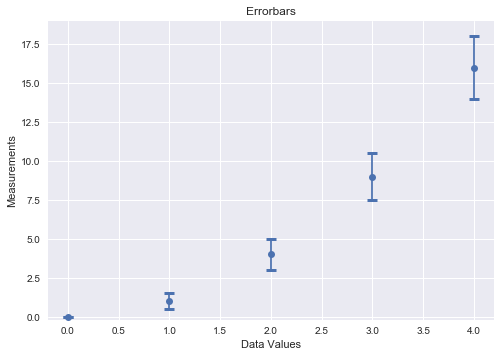## Check for Normality¶

In :
# Visual check
x = randn(100)
_ = stats.probplot(x, plot=plt)
title('Probplot - check for normality')

Out:
<matplotlib.text.Text at 0x24320df7438>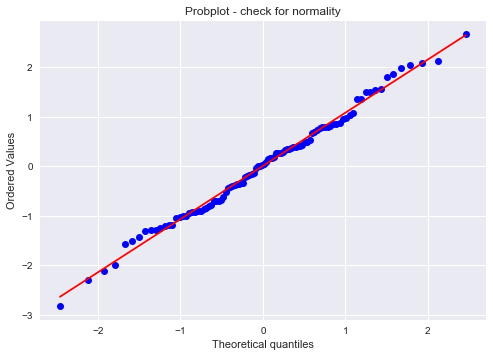## 2D Plot¶

In :
# Generate data
x = randn(200)
y = 10+0.5*x+randn(len(x))

# Scatter plot
scatter(x,y)
# This one is quite similar to "plot(x,y,'.')"
title('Scatter plot of data')
xlabel('X')
ylabel('Y')

Out:
<matplotlib.text.Text at 0x24320e67dd8>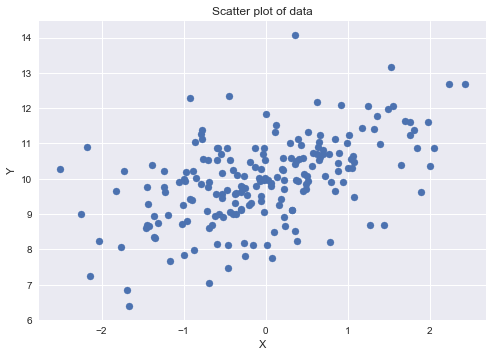## LineFit¶

In :
M = vstack((ones(len(x)), x)).T
pars = linalg.lstsq(M,y)
intercept = pars
slope = pars
scatter(x,y)
plot(x, intercept + slope*x, 'r')
show()